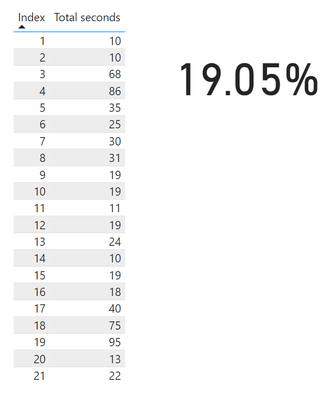cancel
Showing results for
Did you mean:Frequent Visitor

## Help with command

Hello,

I need a command for the following column that will show the percentage of cells higher than 00:00:60 (60 seconds). I appreciate any help.1 ACCEPTED SOLUTIONHelper II

@rigginb , you need to convert to seconds first, then using below measure to calculate, not sure whether my understanding is correct or not, please let me know.
I created below example for 21 rows and 4 out of 21 are greater than 60, that's why 4/21 = 19%

_percentage =
Sheet1,
"@greatthan60",IF(CALCULATE(sum(Sheet1[Total seconds]))>60,1,0)
)
VAR _countgreatthan60 = SUMX(_table,[@greatthan60])
VAR _total_row_count = COUNTROWS(Sheet1)
RETURN
divide(_countgreatthan60,_total_row_count)Helper II

@rigginb , you need to convert to seconds first, then using below measure to calculate, not sure whether my understanding is correct or not, please let me know.
I created below example for 21 rows and 4 out of 21 are greater than 60, that's why 4/21 = 19%

_percentage =
Sheet1,
"@greatthan60",IF(CALCULATE(sum(Sheet1[Total seconds]))>60,1,0)
)
VAR _countgreatthan60 = SUMX(_table,[@greatthan60])
VAR _total_row_count = COUNTROWS(Sheet1)
RETURN
divide(_countgreatthan60,_total_row_count)